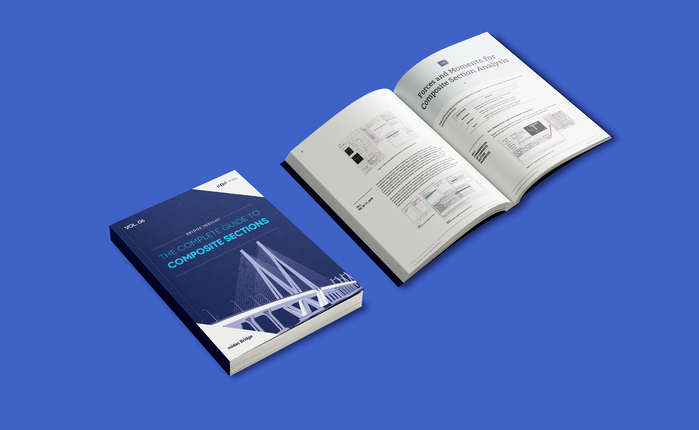BLOG BRIDGE INSIGHT

## Significance of Influence Line Diagram

1. Introduction

2. Considering the rebar confinement effect

3. Application of rebar confinement in midas Civil

4. Conclusion

## 1. Introduction

Concrete shrinks as the cement paste harden. The reinforcements in the section will also serve as a kind of restraint source to prevent concrete from shrinking. For a cantilever rectangular concrete beam, we do not expect a secondary effect. However, if we consider that shrinkage is just happening in concrete and not in reinforcement, then the reinforcement would restrain (confine) the concrete from freely deforming. we can consider the effects restrained by reinforcement for the creep and shrinkage in midas civil

The influence of rebar can be considered in Midas civil for linear and nonlinear analysis. We can define the reinforcement in the section manager for linear analysis and verify the results. We can use fiber material properties to analyse the inelastic time history. In this blog, we are concentrating on linear analysis.

## 2. Considering the rebar confinement effect

Shrinkage depends on many other factors related to the quality of the concrete and conditions of exposure such as the maximum size of aggregate; type of cement; the amount of cement paste; the notional size of concrete members; the amount of steel reinforcement; and curing conditions.

To consider the effect of restraint on creep and shrinkage in the PSC section we need to follow two steps.

a. Click on the Check box on the consider reinforcement for section stiffness calculation in Analysis tab> Main control data as shown in fig 1.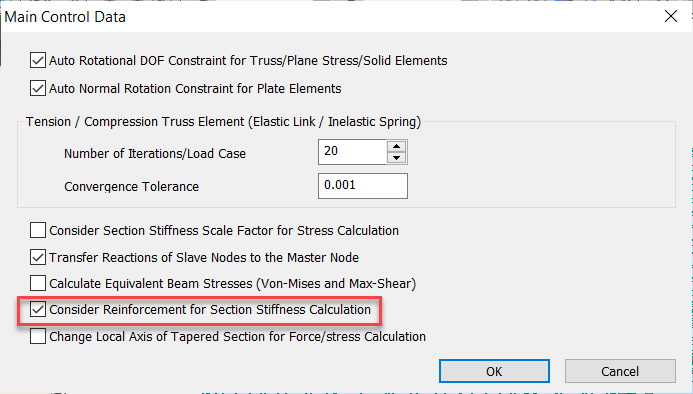Fig1. Main control data dialog box

b. Click on the check box for Consider Re-bar Confinement Effect in Construction stage analysis control data as shown in Figure 2.

Fig2. Consider rebar confinement effect.

3. Application of rebar confinement in midas civil

To explain the rebar confinement effect, I have considered an example of a cantilever beam along with the construction stage analysis. We can reinforce the section to analyze the restraining effect of the rebar. I have compared the models with and without the rebar confinement effect.
The dimensions considered are
Depth = 1000 mm
Width = 1000 mm
Span length = 10m
The eccentricity of the reinforcement = 400 mm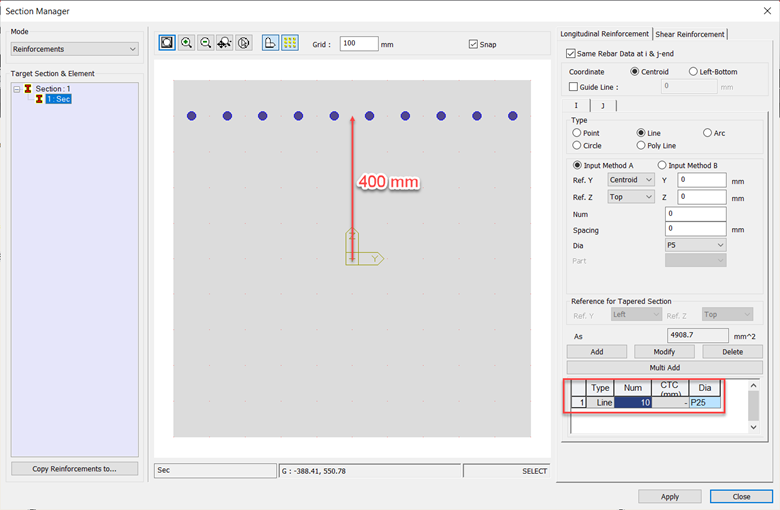Fig3. Section with the rebar arrangement.

Manual Calculation for the shrinkage secondary effect
Fx = - shrinkage strain x Es x As
Es = Modulus of elasticity of steel = 200000 MPa
As = Area of reinforcing steel =4908.7 mm2

The cumulative shrinkage strain is shown in the table below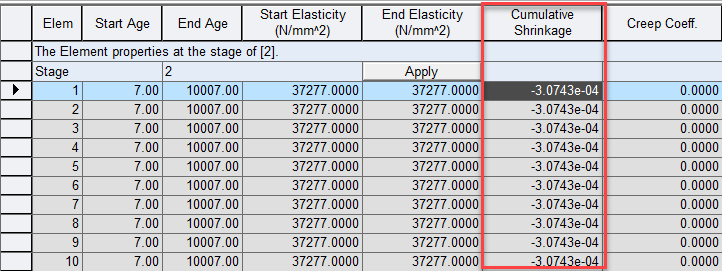Fig4. Cumulative shrinkage properties of the beam.

Shrinkage strain = -3.0743e-04
Axial force (Fx)
Fx = 3.0743e-04 x 200000 x 4908.7
= 301816 N
= 301.8 kN

Bending moment (My)
My = Fx * eccentricity
= 301.8 x 0.4
= 120.7 KN-m

After performing the analysis in the midas Civil, the values of axial force for shrinkage secondary in construction stage 2 for the same model are compared without and with the rebar confinement effect, as shown in fig 4 & 5.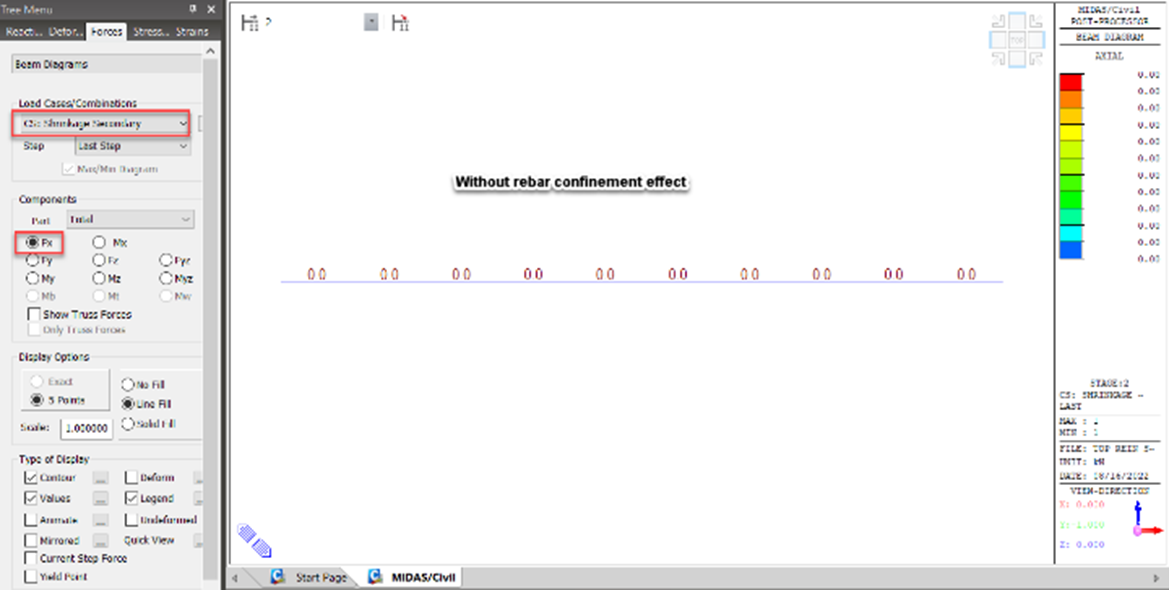Fig.5: Axial force without rebar confinement effect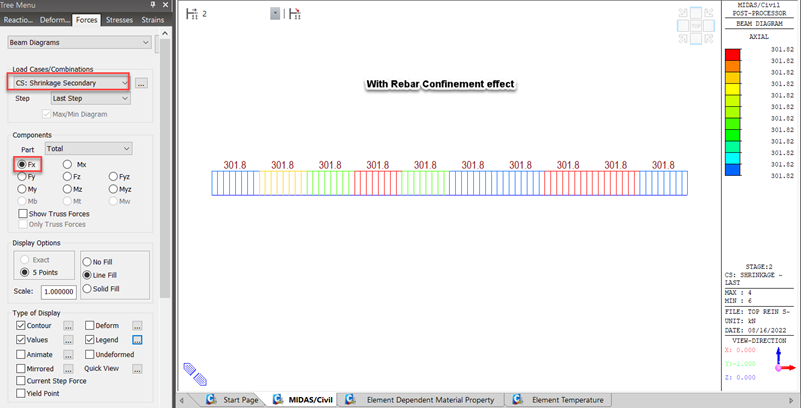Fig6. Axial force with rebar confinement effect

The value of bending moment [My] in the construction stage 2 without and with rebar confinement effect for the shrinkage secondary is shown in figs 6 & 7 respectively.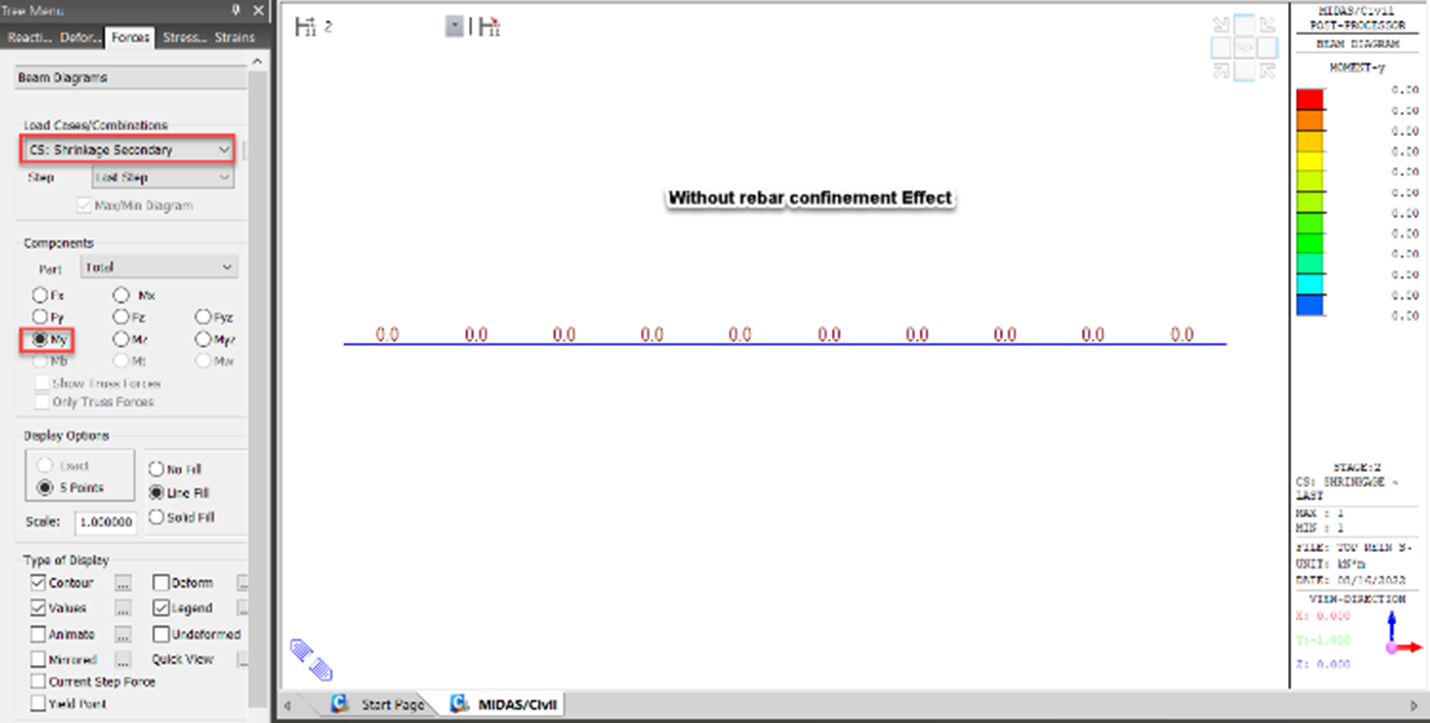Fig.7. Bending moment without rebar confinement effect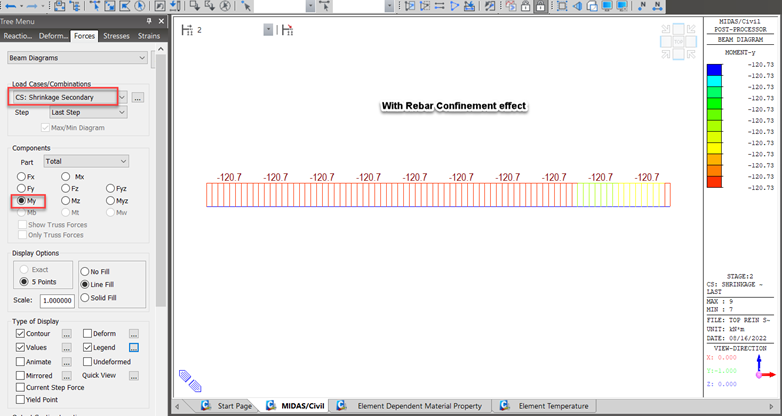Fig.8: Bending moment with rebar confinement effect

4. Conclusion

We have seen significant forces and moments in the concrete member due to the restraining effect of reinforcing bars. Therefore, for considering the restraining effect due to the reinforcement, we can use the function of the rebar confinement effect and consider the reinforcement for section stiffness calculation available in the midas civil.